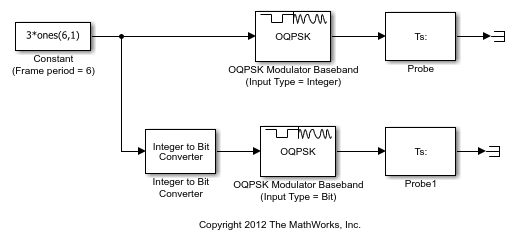Main Content

# Single-Rate Processing with OQPSK Modulator Block

This example shows the OQPSK Modulator Baseband block configured for single-rate processing using integer or bit for the input type.

Use the Open model button to open the `slex_oqpskmod_framebased`> model.In single-rate processing mode, the input and output signals have the same port sample time. The block implicitly implements the rate change by making a size change at the output when compared to the input. In this mode, the output of the block is an integer multiple of the Samples per symbol parameter.

• When you set the Input type parameter to `Integer`, the input can be a scalar value or column vector, the length of which is the number of input symbols.

• When you set the Input type parameter to `Bit`, the input width must be an integer multiple of two.

The output sample period is the period of each integer or bit pair at the input divided by the Samples per symbol.

## Support

#### Bridging Wireless Communications Design and Testing with MATLAB

Download white paper